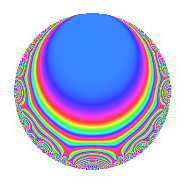# Properties

 Label 134.2.aLevel 134 Weight 2 Character orbit a Rep. character $$\chi_{134}(1,\cdot)$$ Character field $$\Q$$ Dimension 6 Newforms 2 Sturm bound 34 Trace bound 2

# Learn more about

## Defining parameters

 Level: $$N$$ = $$134 = 2 \cdot 67$$ Weight: $$k$$ = $$2$$ Character orbit: $$[\chi]$$ = 134.a (trivial) Character field: $$\Q$$ Newforms: $$2$$ Sturm bound: $$34$$ Trace bound: $$2$$ Distinguishing $$T_p$$: $$3$$

## Dimensions

The following table gives the dimensions of various subspaces of $$M_{2}(\Gamma_0(134))$$.

Total New Old
Modular forms 19 6 13
Cusp forms 16 6 10
Eisenstein series 3 0 3

The following table gives the dimensions of the cuspidal new subspaces with specified eigenvalues for the Atkin-Lehner operators and the Fricke involution.

$$2$$$$67$$FrickeDim.
$$+$$$$-$$$$-$$$$3$$
$$-$$$$+$$$$-$$$$3$$
Plus space$$+$$$$0$$
Minus space$$-$$$$6$$

## Trace form

 $$6q$$ $$\mathstrut +\mathstrut 4q^{3}$$ $$\mathstrut +\mathstrut 6q^{4}$$ $$\mathstrut +\mathstrut 2q^{6}$$ $$\mathstrut +\mathstrut 8q^{9}$$ $$\mathstrut +\mathstrut O(q^{10})$$ $$6q$$ $$\mathstrut +\mathstrut 4q^{3}$$ $$\mathstrut +\mathstrut 6q^{4}$$ $$\mathstrut +\mathstrut 2q^{6}$$ $$\mathstrut +\mathstrut 8q^{9}$$ $$\mathstrut -\mathstrut 6q^{10}$$ $$\mathstrut -\mathstrut 4q^{11}$$ $$\mathstrut +\mathstrut 4q^{12}$$ $$\mathstrut +\mathstrut 8q^{13}$$ $$\mathstrut -\mathstrut 8q^{15}$$ $$\mathstrut +\mathstrut 6q^{16}$$ $$\mathstrut -\mathstrut 6q^{17}$$ $$\mathstrut -\mathstrut 8q^{18}$$ $$\mathstrut +\mathstrut 12q^{19}$$ $$\mathstrut -\mathstrut 12q^{21}$$ $$\mathstrut -\mathstrut 2q^{22}$$ $$\mathstrut -\mathstrut 14q^{23}$$ $$\mathstrut +\mathstrut 2q^{24}$$ $$\mathstrut +\mathstrut 4q^{25}$$ $$\mathstrut -\mathstrut 14q^{26}$$ $$\mathstrut -\mathstrut 8q^{27}$$ $$\mathstrut -\mathstrut 12q^{29}$$ $$\mathstrut -\mathstrut 16q^{30}$$ $$\mathstrut +\mathstrut 16q^{31}$$ $$\mathstrut -\mathstrut 32q^{33}$$ $$\mathstrut -\mathstrut 20q^{35}$$ $$\mathstrut +\mathstrut 8q^{36}$$ $$\mathstrut +\mathstrut 4q^{37}$$ $$\mathstrut -\mathstrut 16q^{39}$$ $$\mathstrut -\mathstrut 6q^{40}$$ $$\mathstrut -\mathstrut 4q^{41}$$ $$\mathstrut +\mathstrut 4q^{43}$$ $$\mathstrut -\mathstrut 4q^{44}$$ $$\mathstrut -\mathstrut 12q^{45}$$ $$\mathstrut +\mathstrut 8q^{46}$$ $$\mathstrut +\mathstrut 22q^{47}$$ $$\mathstrut +\mathstrut 4q^{48}$$ $$\mathstrut +\mathstrut 22q^{49}$$ $$\mathstrut +\mathstrut 8q^{50}$$ $$\mathstrut +\mathstrut 8q^{51}$$ $$\mathstrut +\mathstrut 8q^{52}$$ $$\mathstrut -\mathstrut 12q^{53}$$ $$\mathstrut +\mathstrut 20q^{54}$$ $$\mathstrut +\mathstrut 16q^{55}$$ $$\mathstrut +\mathstrut 20q^{57}$$ $$\mathstrut -\mathstrut 12q^{58}$$ $$\mathstrut -\mathstrut 8q^{60}$$ $$\mathstrut +\mathstrut 36q^{61}$$ $$\mathstrut +\mathstrut 8q^{62}$$ $$\mathstrut -\mathstrut 20q^{63}$$ $$\mathstrut +\mathstrut 6q^{64}$$ $$\mathstrut +\mathstrut 12q^{65}$$ $$\mathstrut +\mathstrut 8q^{66}$$ $$\mathstrut -\mathstrut 6q^{68}$$ $$\mathstrut +\mathstrut 16q^{69}$$ $$\mathstrut +\mathstrut 20q^{70}$$ $$\mathstrut +\mathstrut 14q^{71}$$ $$\mathstrut -\mathstrut 8q^{72}$$ $$\mathstrut -\mathstrut 14q^{73}$$ $$\mathstrut -\mathstrut 4q^{74}$$ $$\mathstrut +\mathstrut 64q^{75}$$ $$\mathstrut +\mathstrut 12q^{76}$$ $$\mathstrut +\mathstrut 8q^{77}$$ $$\mathstrut +\mathstrut 4q^{78}$$ $$\mathstrut +\mathstrut 4q^{79}$$ $$\mathstrut +\mathstrut 6q^{81}$$ $$\mathstrut +\mathstrut 4q^{82}$$ $$\mathstrut -\mathstrut 4q^{83}$$ $$\mathstrut -\mathstrut 12q^{84}$$ $$\mathstrut -\mathstrut 28q^{85}$$ $$\mathstrut +\mathstrut 2q^{86}$$ $$\mathstrut -\mathstrut 12q^{87}$$ $$\mathstrut -\mathstrut 2q^{88}$$ $$\mathstrut +\mathstrut 22q^{89}$$ $$\mathstrut -\mathstrut 42q^{90}$$ $$\mathstrut +\mathstrut 16q^{91}$$ $$\mathstrut -\mathstrut 14q^{92}$$ $$\mathstrut -\mathstrut 20q^{93}$$ $$\mathstrut +\mathstrut 20q^{94}$$ $$\mathstrut +\mathstrut 2q^{96}$$ $$\mathstrut +\mathstrut 16q^{97}$$ $$\mathstrut -\mathstrut 16q^{98}$$ $$\mathstrut -\mathstrut 32q^{99}$$ $$\mathstrut +\mathstrut O(q^{100})$$

## Decomposition of $$S_{2}^{\mathrm{new}}(\Gamma_0(134))$$ into irreducible Hecke orbits

Label Dim. $$A$$ Field CM Traces A-L signs $q$-expansion
$$a_2$$ $$a_3$$ $$a_5$$ $$a_7$$ 2 67
134.2.a.a $$3$$ $$1.070$$ 3.3.473.1 None $$-3$$ $$1$$ $$3$$ $$0$$ $$+$$ $$-$$ $$q-q^{2}+\beta _{2}q^{3}+q^{4}+(1+\beta _{1})q^{5}-\beta _{2}q^{6}+\cdots$$
134.2.a.b $$3$$ $$1.070$$ $$\Q(\zeta_{18})^+$$ None $$3$$ $$3$$ $$-3$$ $$0$$ $$-$$ $$+$$ $$q+q^{2}+(1+\beta _{1})q^{3}+q^{4}+(-1-\beta _{1}+\cdots)q^{5}+\cdots$$

## Decomposition of $$S_{2}^{\mathrm{old}}(\Gamma_0(134))$$ into lower level spaces

$$S_{2}^{\mathrm{old}}(\Gamma_0(134)) \cong$$ $$S_{2}^{\mathrm{new}}(\Gamma_0(67))$$$$^{\oplus 2}$$